Function Repository Resource:

# FourierShift

Shift the zero-frequency term to the center of the spectrum

Contributed by: Yong-an Lu
 ResourceFunction["FourierShift"][data] rearranges a Fourier transform data by shifting the zero-frequency term to the center of the array. ResourceFunction["FourierShift"][data,dim] operates along the dimension dim of data.

## Details and Options

In optics, the zero-frequency term appears in the center of spectrum.
For Fourier, the zero-frequency term appears at position 1 in the resulting list; ResourceFunction["FourierShift"] moves it to the middle.

## Examples

### Basic Examples (4)

Swap the left and right halves of a vector:

 In:=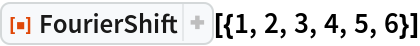Out=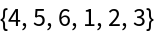If a vector has an odd number of elements, then the shift moves the first element to the center:

 In:=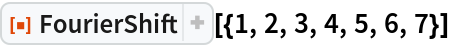Out=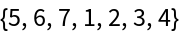Swap the first quadrant of the matrix with the third, and the second quadrant with the fourth:

 In:=Out=Swap halves of each column of matrix:

 In:=Out=Swap halves of each row instead:

 In:=Out=### Scope (2)

FourierShift can be applied to a multidimensional array:

 In:=Out=The dimension can be any valid part specification:

 In:=Out=In:=Out=### Properties and Relations (2)

For Fourier, the zero-frequency term appears at position 1 in the resulting list:

 In:=Out=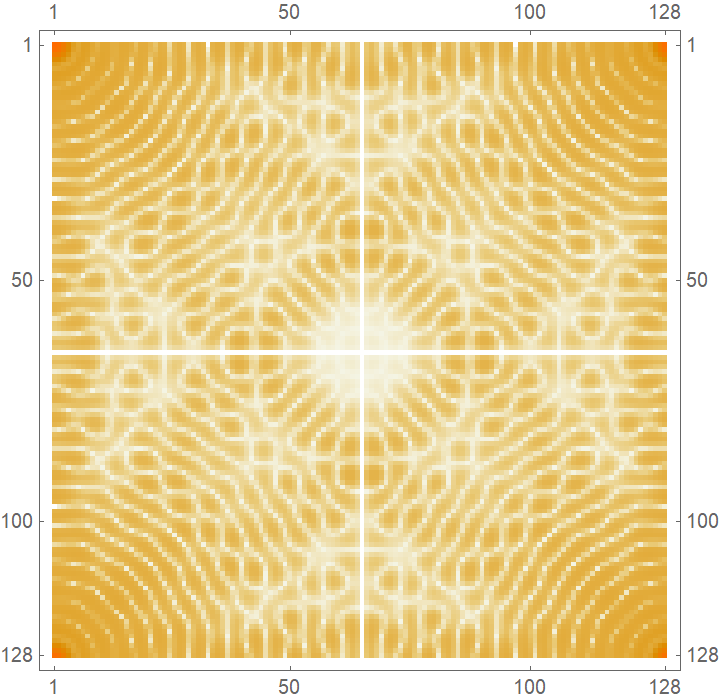FourierShift shifts zero-frequency term to center of spectrum:

 In:=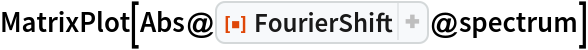Out=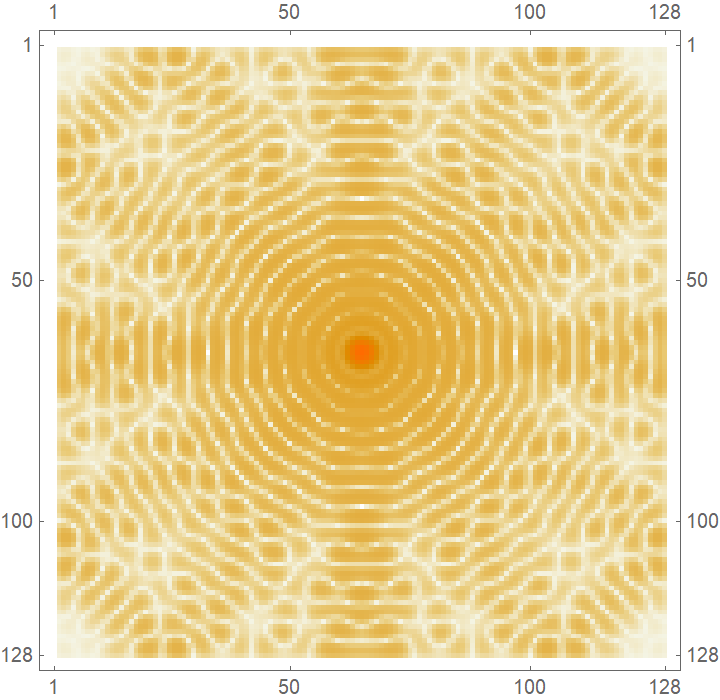### Possible Issues (2)

FourierShift does not support ragged arrays:

 In:=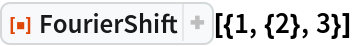Out=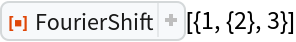Calling FourierShift twice does not necessarily reconstruct the original input:

 In:=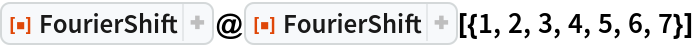Out=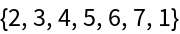Yong-an Lu

## Version History

• 1.0.0 – 10 October 2019